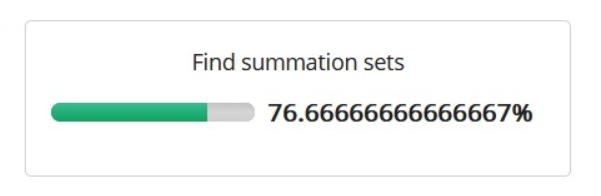## Question :

Consider a non-empty array of integers inarr with the elements separated by “,”(comma) and an integer innum. Find and print a number outnum based on the below logic:

Identify all possible unique combinations of four elements from inarr A unique combination is one where, irrespective of the order, the same set of elements in the combination will not appear in any other combination

. If there are one or more combinations whose sum of elements is equal to innum, set outnum with the count of combinations

. If there is no combination whose sum is equal to innum, print -1

Assumption:

. inarr will contain at least 4 elements

## Question :## Code Solution :

```				```
def solve(s,t):
arr=s
for x in range(len(arr)):
arr[x]=int(arr[x])
count=0
n=len(arr)
for i in range(n):
for j in range(i+1,n):
for k in range(j+1,n):
for l in range(k+1,n):
sum = arr[i]+arr[j]+arr[k]+arr[l]
if sum == t:
count=count+1
if count==0:
return -1
else:
return count

s=input().split(",")
p=int(input())
print(solve(s,p))
```
```